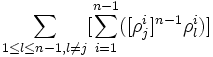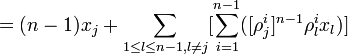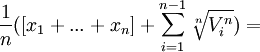## Friday, January 11, 2008

### Vandermonde: Vandermonde Equation

Alexandre-Theophile Vandermonde independently discovered what is today called the Lagrange Resolvent. Some mathematical historians have politely called it the Lagrange (Vandermonde) Resolvent. Even so, there is little doubt that Vandermonde discovered the idea independently of Joseph-Louis Lagrange and only Vandermonde applied the idea to solve the eleventh root of unity. The Lagrange Resolvent is the equation:where

ρ1, ..., ρn are n of the n-th roots of unity.

Below I present the reasoning that led Vandermonde to the above formula:

Vandermonde sought to find a function on n values that returned its answer those same n different values. In other words, a function of n values that returns the n values themselves as the answer.

If we are only talking about two values, then such an equation is pretty easy to find:

F(x1, x2) = (1/2)[(x1 + x2) + √(x1 - x2)2

To get two possible answers, we use the (x1 - x2)2. This has two possible answers ± (x1 - x2)

The two results then are:

(1/2)[(x1 + x2) + x1 - x2)] = (1/2)[2*x1] = x1

and

(1/2)[(x1 + x2) + -(x1 - x2)] = (1/2)[x1 + x2 -x1 + x2] = (1/2)[2*x2] = x2

Vandermonde was able to find an equation that worked for an equation of n values which I present below.

Lemma 1:

if l1]i = [ρl2]i where:

ρl is an n-th root of unity and 1 ≤ i ≤ n-1 and 1 ≤ l1,l2 ≤ n-1

Then:

l1 = l2

Proof:

(1) Assume that l1 ≠ l2

(2) Then l1]i ≠ [ρl2]i [since each of the ρl are distinct]

(3) But this is not the case so we reject our assumption in step #1.

QED

Lemma 2:

In the sum:where ρ1, ..., ρn are n of the n-th roots of unity.

It follows that each product above is an n-th root of unity and further, each product represents a distinct n-th root of unity ≠ 1

Proof:

(1) Now: [(ρj)i]n-1*(ρl)i is itself an n-th root of unity, since:

{[(ρj)i]n-1*(ρl)i}n = [(ρj)n]i(n-1)*[(ρl)n]i =

= (1)i(n-1)*(1)i = 1

(2) Assume that [(ρj)i]n-1*(ρl)i = 1

(3) Now j)n-1*(ρj) = 1 so that:

[(ρj)n-1*(ρj)]i = 1

and

[(ρj)n-1]i*(ρj)i = 1

(4) So,[(ρj)n-1]i*(ρj)i= [(ρj)i]n-1*(ρl)i

(5) Which gives us that:

j]i = [ρl]i which is impossible since l ≠ j. [See Lemma 1 above]

(6) So we have a contradictoin and reject our assumptoin at step #2.

(7) Assume that each product is not distinct so that there exists two products such that:

[(ρj)i]n-1*(ρl1)i = [(ρj)i]n-1*(ρl2)i

where l1 ≠ l2.

(8) Then it follows that:

l1)i = (ρl2)i .

(9) But this is impossible since l1 ≠ l2. [See Lemma 1 above]

(10) So, we have a contradiction and we reject our assumption in step #7.

QED

Theorem 3: Vandermonde Equation

If:whereand

ρ1, ..., ρn are n of the n-th roots of unity.

Then:

this equation returns x1, ..., xn in its set of possible values

Proof:

(1) First, we note that there are n possible roots for:which are:

ρ1*Vi, ρ2*Vi, ..., ρn*Vi

This follows since: k*Vi)n = (ρk)n*(Vi)n = (1)*(Vi)n = (Vi)n

(2) Let's consider that we want to return xj as an answer.

(3) Consider ρk*Vi such that ρk = ([ρj]i)n-1

Note: We know that ([ρj]i)n-1 is a root of unity since:

[([ρj]i)n-1]n = [(ρj)n]i*[n-1] = (1)i*[n-1] = 1

(4) So, we have:

ρk*Vi = ([ρj]i)n-1*[Vi] =(5) And:(6) Now, combining step #5 with the main equation gets us:(7) Using Lemma 2 above, we know that each of the n-1 n-th root of unity products is distinct and ≠ 1.

(8) From step #7, it follows that there is a mapping from each product to a distinct ρm

Thus, we have:where ρm ≠ 1.

(9) Now, since each n-th root of unity is itself a power of a primitive n-root of unity (see Theorem 3, here), if we let ρ be any primitive n-th root of unity, then we have:(10) Now, from a previous result (see Lemma 1, here), we know that:

1 + ρ + ρ2 + ... + ρn-1 = 0

(11) So, now we can greatly simplify the equation in step #6:QED

References
• Jean-Pierre Tignol, Galois' Theory of Algebraic Equations, World Scientific, 2001